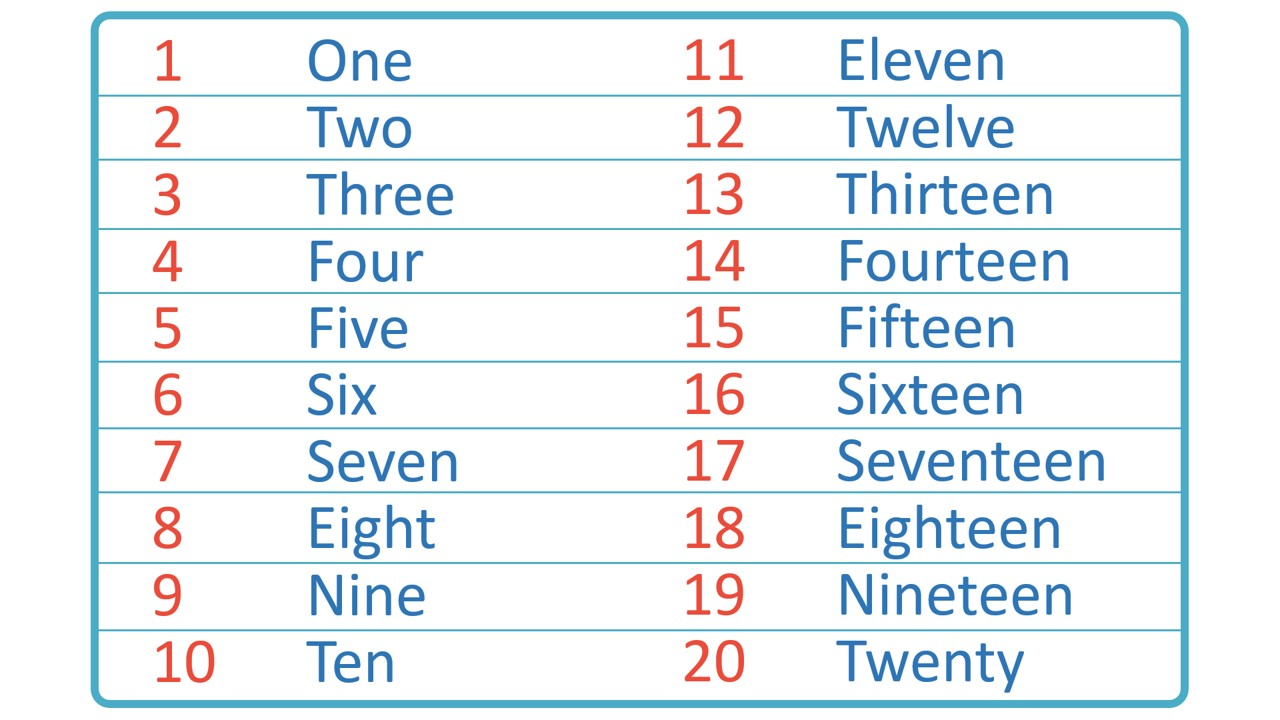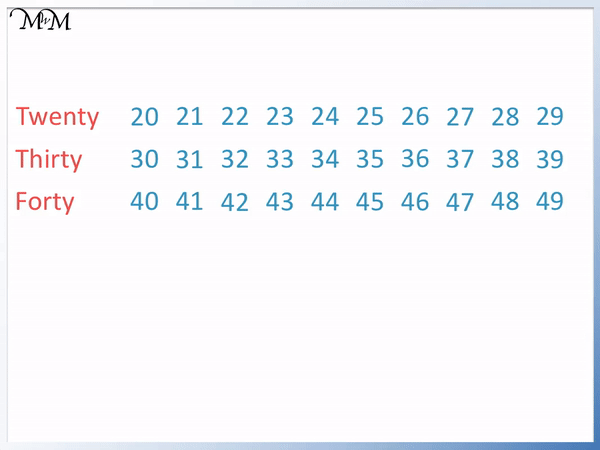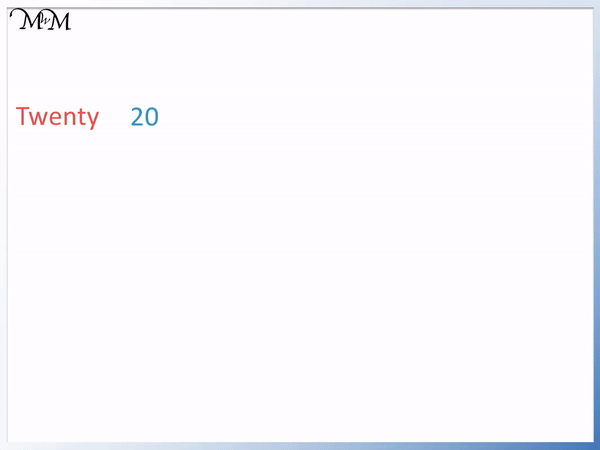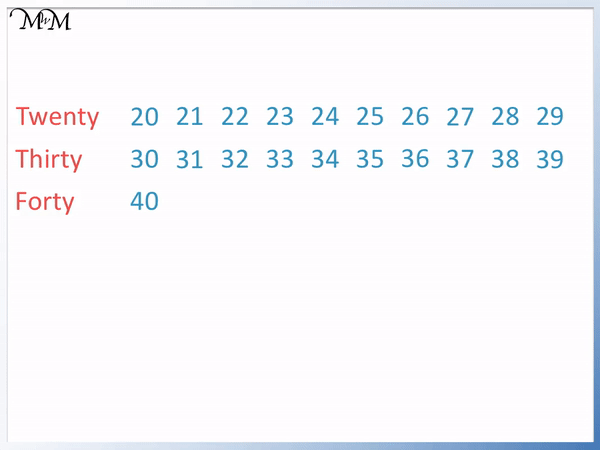# Writing Numbers to 100 in Words

Writing Numbers to 100 in WordsHere are the numbers 1 to 20:• There is a pattern to reading numbers that are larger than 20.
• The tens digit tells us which multiple of ten we are working in.
• The units digit is simply read after this multiple of ten.
• We put a hyphen ‘-‘ between the multiple of ten and the number represented by the units digit.

If a number is greater than 20, first read the multiple of ten that we are working in and then read the units digit afterwards.#### Accompanying Activity Lesson Worksheet# How to Read and Write Numbers to 100 in Words

Here are the numbers from one to twenty written in words.Before teaching reading numbers to 100 in words, it is first important to be familiar with the numbers to twenty.

We will continue by looking at the pattern in reading the numbers from 20 to 100.

To read numbers to 100 in words, first read the multiple of ten given by the tens digit and then read the units digit immediately afterwards. There should always be a hyphen ‘-‘ separating the multiple of ten and the number given by the units digit. The numbers ‘one’ to ‘nine’ repeat, written after each new multiple of ten.

Here is a list of the multiples of ten written in words:

• 20 – Twenty
• 30 – Thirty
• 40 – Forty
• 50 – Fifty
• 60 – Sixty
• 70 – Seventy
• 80 – Eighty
• 90 – Ninety

We will first learn how to read numbers in the twenties in words.

20 is the number twenty. All 2-digit whole numbers that start with a ‘2’ digit are in the twenties.21 starts with a ‘2’ and so it is in the twenties. Therefore we first write ‘twenty’.

21 ends in a ‘1’ in the units column and so we simply write a ‘one’ after the ‘twenty’.

21 is written in words as ‘twenty-one’. We put a hyphen between twenty and one.

22 is written as ‘twenty-two’.

23 is written as ‘twenty-three’

24 is written as ‘twenty-four’.

25 is written as ‘twenty-five’

26 is written as ‘twenty-six’.

27 is written as ‘twenty-seven’

28 is written as ‘twenty-eight’.

29 is written as ‘twenty-nine’

We can see that as long as we know the numbers from 1 to 9, we simply put a ‘twenty’ in front of them to write numbers in the twenties.

30 comes after 29.

30 is read as ‘thirty’ and is the first 2-digit number that starts with a 3.

Here we will read and write the numbers in the thirties. The numbers in the thirties are the 2-digit whole numbers that start with a 3.31 starts with a ‘3’ and so it is in the thirties. Therefore we first write ‘thirty’.

31 ends in a ‘1’ in the units column and so we simply write a ‘one’ after the ‘thirty’.

31 is written in words as ‘thirty-one’. We put a hyphen between thirty and one.

32 is written as ‘thirty-two’.

33 is written as ‘thirty-three’

34 is written as ‘thirty-four’.

35 is written as ‘thirty-five’

36 is written as ‘thirty-six’.

37 is written as ‘thirty-seven’

38 is written as ‘thirty-eight’.

39 is written as ‘thirty-nine’

Again, we simply repeat the numbers 1 to 9 but with the word ‘thirty’ in front of them. The thirty and the units number are separated by a hyphen.

40 comes after 39.

40 is read as ‘forty’. It is a common mistake to spell forty as fourty, with a ‘u’, like the number four. This is incorrect and forty is spelled without a ‘u’.

All 2-digit whole numbers that start with a ‘4’ are in the forties.

They start with the word ‘forty’, are followed by a hyphen and then the units number.41 starts with a ‘4’ and so it is in the forties. Therefore we first write ‘forty’.

41 ends in a ‘1’ in the units column and so we simply write a ‘one’ after the ‘forty’.

41 is written in words as ‘forty-one’. We put a hyphen between forty and one.

42 is written as ‘forty-two’.

43 is written as ‘forty-three’

44 is written as ‘forty-four’.

45 is written as ‘forty-five’

46 is written as ‘forty-six’.

47 is written as ‘forty-seven’

48 is written as ‘forty-eight’.

49 is written as ‘forty-nine’

We can see that the rule learnt previously continues.

We simply write the multiple of ten indicated by the first digit and follow this with a hyphen and then the final digit.

After forty, the next multiples of ten are fifty (50), sixty (60), seventy (70), eighty (80) and ninety (90).The numbers continue to end in ‘one’, ‘two’, ‘three’, ‘four’, ‘five’, ‘six’, ‘seven’, eight’ and ‘nine’.

The last number in this pattern is 99, which is written as ‘ninety-nine’.

After ninety-nine comes one hundred, 100.Now try our lesson on Ordering Numbers to 100 where we learn how to order the numbers to 100.